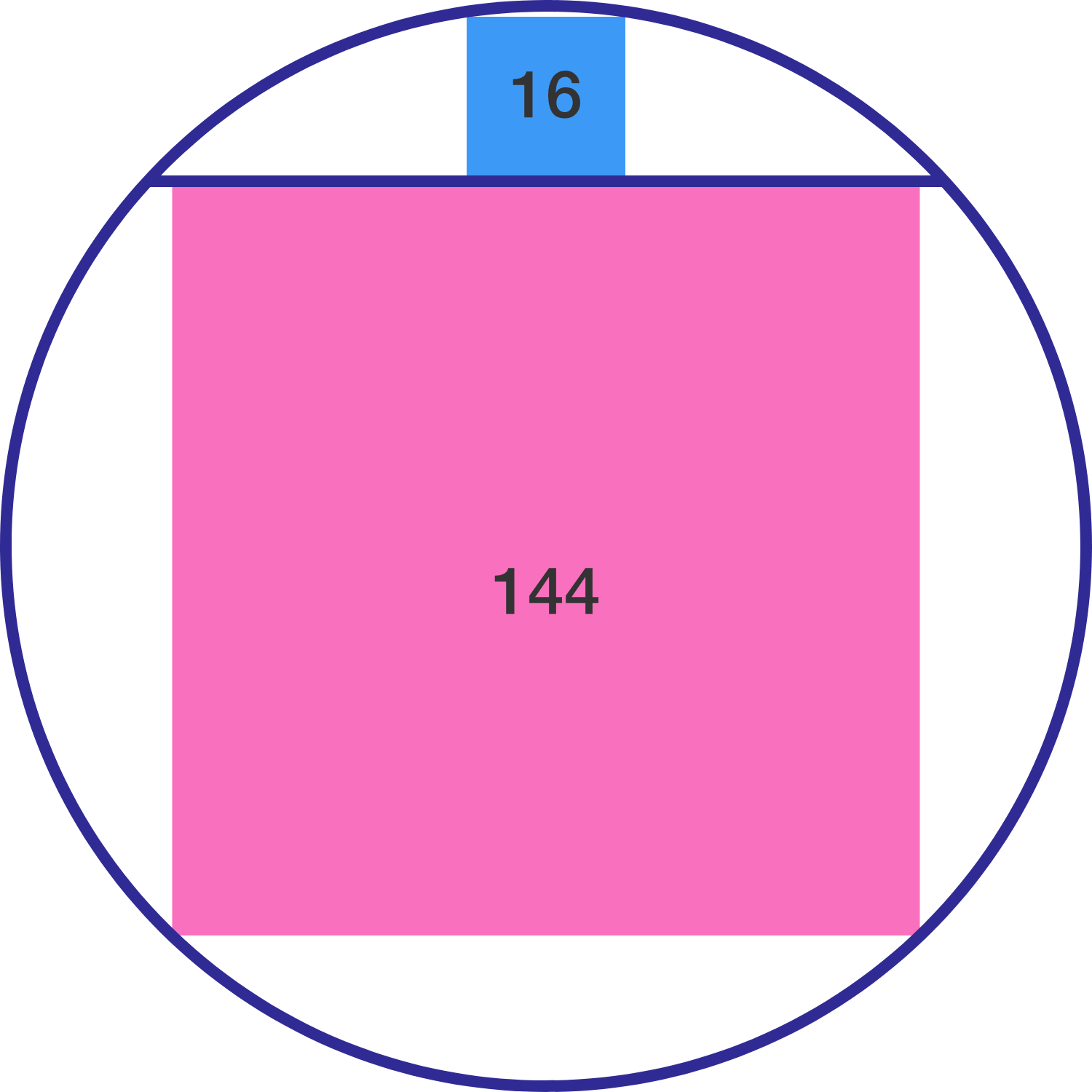Squares in circleA chord of a circle divides the circle into two parts such that the squares inscribed in the two parts have areas 16 and 144, respectively.

The radius of the circle is $\text{\_\_\_\_\_\_\_\_\_\_}.$

×